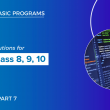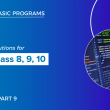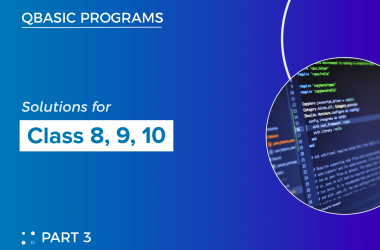# Qbasic programs solutions(part 8) for class 8, class 9 and class 10

Here, I have listed some of the qbasic programs. We also have mobile app where you can view all the qbasic programs. For better experience I highy recommend you to use our mobile app

141  Write a program in qbasic TO CHECK WHETHER THE ASK NUMBER IS PERFECT SQUARE NUMBER OR NOT

``````CLS
INPUT "ENTER ANY NUMBER"; N
S = SQR(N)
IF S = INT(S) THEN
PRINT "PERFECT SQUARE"
ELSE
PRINT "NOT PERFECT SQUARE"
END IF
END ``````

142  Write a program to input sales amount and rate of commission then calculate commission and return net sales. [ns=sa-c)

``````REM PROGRAM TO CALCULATE NET SALES
CLS
INPUT “ENTER SALES AMOUNT”; A
INPUT “ENTER COMMISSION RATE”; R
C = R / 100 * A
NS = A - C
PRINT “NET SALES=”; NS
END ``````

143  Write a program in qbasic to print all odd numbers from 1 to 100 also print its sum

``````CLS
FOR I = 1 TO 100 STEP 2
PRINT I,
S = S + I
NEXT I
PRINT “SUM OF ALL ODD NUMBERS FROM 1 TO 100=”; S
END ``````

144  Write a program in qbasic to enter any three strings and print the longest one

``````CLS
INPUT "ENTER FIRST STRING"; A\$
INPUT "ENTER SECOND STRING"; B\$
INPUT "ENTER THIRD STRING"; C\$
IF LEN(A\$) > LEN(B\$) AND LEN(A\$) > LEN(C\$) THEN
G\$ = A\$
IF LEN(B\$) > LEN(A\$) AND LEN(B\$) > LEN(C\$) THEN
G\$ = B\$
ELSE
G\$ = C\$
END IF
PRINT "LONGEST STRING="; G\$
END ``````

145  Write a program in qbasic to print all even numbers from 2 to 100

``````CLS
FOR I = 2 TO 100 STEP 2
PRINT I,
NEXT I
END ``````

146  Write a program in qbasic to ask any string and count total no. of vowels    consonants    words and sentences

``````CLS
INPUT "ENTER ANY STRING"; S\$
VC = 0
CC = 0
WC = 1
SC = 0
FOR I = 1 TO LEN(S\$)
B\$ = MID\$(S\$, I, 1)
C\$ = UCASE\$(B\$)
IF C\$ = "A" OR C\$ = "E" OR C\$ = "I" OR C\$ = "O" OR C\$ = "U" THEN
VC = VC + 1
ELSEIF B\$ = " " THEN
WC = WC + 1
ELSEIF B\$ = "." THEN
SC = SC + 1
ELSE
CC = CC + 1
END IF
NEXT I
PRINT "TOTAL NO. OF VOWELS= "; VC
PRINT "TOTAL NO. OF CONSONANTS="; CC
PRINT "TOTAL NO. OF WORDS="; WC
PRINT "TOTAL NO. OF SENTENCES="; SC
END ``````

147  Write a program in qbasic to generate the following series 11   3   14   17   31…………18th term

``````CLS
A = 11
B = 3
FOR I = 1 TO 18
PRINT A;
C = A + B
A = B
B = C
NEXT I
END``````

148  Write a program in qbasic to print sum of cube of any three ask numbers

``````REM PROGRAM TO DISPLAY SUM OF CUBE OF ANY THREE INPUT NUMBERS
CLS
INPUT “ENTER FIRST NUMBER”; A
INPUT “ENTER SECOND NUMBER”; B
INPUT “ENTER THIRD NUMBER”; C
S = A ^ 3 + B ^ 3 + C ^ 3
PRINT “SUM OF CUBE OF THREE NUMBERS ”; S
END
﻿``````

149  Write a program in qbasic to enter any 10 numbers and sort in ascending order

``````CLS
DIM N(10)
FOR I = 1 TO 10
INPUT "ENTER THE NUMBERS"; N(I)
NEXT I
FOR I = 1 TO 10
FOR J = 1 TO 10 - I
IF N(J) > N(J + 1) THEN SWAP N(J), N(J + 1)
NEXT J
NEXT I
PRINT "NUMBERS ARRANGED IN ASCENDING ORDER"
FOR I = 1 TO 10
PRINT N(I)
NEXT I
END
﻿``````

150   Write a program in qbasic to ask number and check whether the given number is composite or not

``````CLS
INPUT "ENTER ANY NUMBER"; N
C = 0
FOR I = 1 TO N
IF N MOD I = 0 THEN C = C + 1
NEXT I
IF C <> 2 THEN
PRINT N; "IS COMPOSITE NUMBER"
ELSE
PRINT N; "IS NOT COMPOSITE NUMBER"
END IF
END
﻿``````

151  Write a program in qbasic to enter any three strings and print the shortest one

``````CLS
INPUT "ENTER FIRST STRING"; A\$
INPUT "ENTER SECOND STRING"; B\$
INPUT "ENTER THIRD STRING"; C\$
IF LEN(A\$) < LEN(B\$) AND LEN(A\$) < LEN(C\$) THEN
S\$ = A\$
IF LEN(B\$) < LEN(A\$) AND LEN(B\$)< LEN(C\$) THEN
S\$ = B\$
ELSE
S\$ = C\$
END IF
PRINT "SHORTEST STRING="; S\$
END ``````

152  Write a program in qbasic to ask number and find product of odd digits

``````CLS
INPUT "ENTER ANY NUMBER"; N
P = 1
WHILE N <> 0
R = N MOD 10
IF R MOD 2 = 1 THEN P = P * R
N = N \ 10
WEND
PRINT "PRODUCT OF ODD DIGITS"; P
END ``````

153  Write a program in qbasic TO ENTER ANY DIGIT AND PRINT EVEN DIGITS

``````CLS
INPUT "ENTER ANY NUMBER"; N
PRINT "EVEN DIGITS ARE ";
WHILE N <> 0
R = N MOD 10
IF R MOD 2 = 0 THEN PRINT R;
N = N \ 10
WEND
END ``````

154  Write a program in qbasic to ask number and find sum of even digits

``````CLS
INPUT "ENTER ANY NUMBER"; N
S = 0
WHILE N < > 0
R = N MOD 10
IF R MOD 2 = 0 THEN S = S + R
N = N \\ 10
WEND
PRINT "SUM OF EVEN DIGITS"; S
END ``````

155  Write a program in qbasic to print the sum of the numbers between 3 to 30

``````CLS
FOR I = 3 TO 30
S = S + I
NEXT I
PRINT “SUM OF NUMBERS BETWEEN 3 TO 30” ; S
END ``````

156  Write a program in qbasic to generate 7   22   11   34……………19th terms

``````CLS
A = 9
FOR I = 1 TO 10
PRINT A;
IF A MOD 2 = 0 THEN
A = A \ 2
ELSE
A = A * 3 + 1
END IF
NEXT I
END  ``````

157  Write a program in qbasic to generate the series 10   12   15   19…………..14th terms

``````CLS
A = 10
B = 2
FOR I = 1 TO 14
PRINT A
A = A + B
B = B + 1
NEXT I
END ``````

158  Write a program in qbasic to ask any string and check whether the first character of a ask string is alphabet number or symbol

``````CLS
INPUT "ENTER ANY STRING"; S\$
A\$ = LEFT\$(S\$, 1)
B = ASC(A\$)
IF B >= 48 AND B <= 57 THEN
CK\$ = "FIRST CHARACTER IS A NUMBER"
ELSEIF B >= 65 AND B <= 90 OR B >= 97 AND B <= 122 THEN
CK\$ = "FIRST CHARACTER IS ALPHABET"
ELSE
CK\$ = "FIRST CHARACTER IS SYMBOL"
END IF
PRINT CK\$
END ``````

159  Write a program in qbasic to ask any string and reverse it

``````CLS
INPUT "ENTER ANY STRING"; S\$
FOR I = LEN(S\$) TO 1 STEP -1
B\$ = MID\$(S\$, I, 1)
W\$ = W\$ + B\$
NEXT I
PRINT "REVERSED STRING IS "; W\$
END ``````

160   Write a program in qbasic to enter any 10 numbers and sort in descending order

``````CLS
DIM N(10)
FOR I = 1 TO 10
INPUT "ENTER THE NUMBERS"; N(I)
NEXT I
FOR I = 1 TO 10
FOR J = 1 TO 10 - I
IF N(J)  < N(J + 1) THEN SWAP N(J), N(J + 1)
NEXT J
NEXT I
PRINT "NUMBERS ARRANGED IN DESCENDING ORDER"
FOR I = 1 TO 10
PRINT N(I)
NEXT I ``````

##### Previous Post## Qbasic programs solutions(part 7) for class 8, class 9 and class 10

##### Next Post## Qbasic programs solutions(part 9) for class 8, class 9 and class 10

##### Related Posts## Qbasic programs solutions(part 3) for class 8, class 9 and class 10

Here, I have listed some of the qbasic programs. We also have mobile app where you can view…## Features of Qbasic Programming language

1. Simple and easy to learn the language: It has been called a “people’s language.” With the help…## Statements in Qbasic:

A statement is a collection of instructions written in QBASIC using keywords and commands. There are three types…